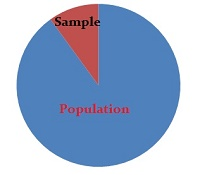# Population Percentage

Share onIt’s unlikely you can survey everyone, so you survey a smaller percentage of the population to get statistics.

The idea of a population percentage often shows up when calculating confidence intervals. It is a type of population parameter , which means that it concerns everyone in the population — not just a sample.

## Population Percentage Example

For example, the population of the United States in 2021 was 331.9 million. Of that total, 5.7% were under 5 years old. That fact is a population percentage.

However, statistics is concerned with samples — not entire populations. Unless you have the means to conduct a survey of the entire population (like a census), you won’t be able to ask everyone how old they are in order to calculate an exact figure (5.7%). Instead, you ask a proportion of the population — say, 5,000 people — how old they are, then you use this information to create a “best guess” about the true population percentage. You might get close — somewhere between 5 and 6% — but it’s very unlikely you’ll get an exact number. Therefore, you create a confidence interval and say that the population percentage of under fives is between 5 and 6%.

## References

CITE THIS AS:
Stephanie Glen. "Population Percentage" From StatisticsHowTo.com: Elementary Statistics for the rest of us! https://www.statisticshowto.com/population-percentage/
---------------------------------------------------------------------------Need help with a homework or test question? With Chegg Study, you can get step-by-step solutions to your questions from an expert in the field. Your first 30 minutes with a Chegg tutor is free!